### CS2201 DATA STRUCTURES Two Mark Questions With Answers 2014

Anna University, Chennai

CS2201 DATA STRUCTURES UNIT –I

LINEAR STRUCTURES

1. What are the main objectives of Data structure?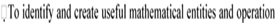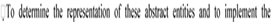problems can be solved by using these entities and operations. operations on these concrete representation.

2. Need of a Data structure?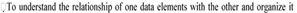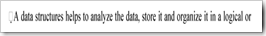memory. manner.

3. Define data structure with example.

s to determine what classes of abstract

within the mathematical

A data structure is a way of organizing data that considers not only the items stored but also their relationship to each other.

e.g.: Arrays, Records etc.

e.g of complex data structure

Stacks, Queues, Linked list, Trees, Graphs.

4. Define abstract data type and list its advantages.

ADT is a set of operations.

An abstract data type is a data declaration packaged together with the operations that are meaning full on the data type.

Abstract Data Type

1. Declaration of data

2. Declaration of operations.

1. Easy to debug small routines than large ones.

2. Easy for several people to work on a modular program simultaneously.

3. A modular program places certain dependencies in only one routine, making changes easier.

5. What are the two basic types of Data structures?

1. Primitive Data structure

Eg., int,char,float

2. Non Primitive Data Structure i. Linear Data structure

Eg., Lists Stacks Queues ii. Non linear Data structure

Eg., Trees Graphs

6. List out the different ways to implement the list?

1. Array Based Implementation

7. Define singly linked list with neat diagram.

A singly linked list is a collection of nodes each node is a structure it consisting of an element and a pointer to a structure containing its successor, which is called a next pointer.

The last cell’s next pointer points to NULL specified by zero.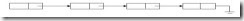8. Write the routine for insertion operation of singly linked list.

Void Insert (ElementType X, List L, Position P)

{ Position TmpCell; TmpCell=malloc(sizeof(struct Node)); if(TmpCell==NULL)

FatalError(“Out of space!!!”); TmpCell->Element =X; TmpCell->Next=P->Next;

P->Next=TmpCell;

}

1. Easy insertion and deletion.

1. Data not present in a linear manner.

2. Insertion and deletion from the front of the list is difficult without the use of header

node.

Doubly linked list is a collection of nodes where each node is a structure containing the following fields

1. Pointer to the previous node.

2. Data.

3. Pointer to the next node.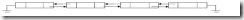11. Define circularly linked list with neat diagram.

Circularly linked list is a collection of nodes , where each node is a structure containing the element and a pointer to a structure containing its successor.

The pointer field of the last node points to the address of the first node. Thus the linked list becomes circular.

12. Define stack ADT with example.

A stack is a list with a restriction that insertions and deletions can be performed in only one position namely the end of the list called the top.

e.g.: undo statement in text editor. Pile of bills in a hotel.

13. State the operations on stack. Define them and give the diagrammatic representation. Push

Pop

Top

Push: Push is performed by inserting at the top of stack.

Pop: pop deletes the most recently inserted element.

Top: top operation examines the element at the top of the stack and returns its value.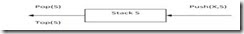14. Write the routine for push operation.

Void Push(ElementType X,Stack S) Stack S

Pop(S) Push(X,S) Top(S)

{

PtrToNode TmpCell; TmpCell=malloc(sizeof(struct Node)); If(TmpCell==NULL)

FatalError(“out of space!!!”);

else{

TmpCell->Element=x; TmpCell->Next=s->Next; S->Next=TmpCell;

}}

15. What is the purpose of top and pop?

Top operation examines the element in the top of the list and returns its value. Pop operation deletes the element at the top of the stack and decrements the top of the stack pointer by one.

1. Calls to malloc and free functions are expensive.

2. Using pointers is expensive.

17. State the applications of stack.

1. Balancing parentheses.

2. Postfix Expression.

i. Infix to postfix conversion

3. Function calls.

18. Convert into postfix and evaluate the following expression.

(a+b*c)/d

a=2 b=4 c=6 d=2

Ans: Post fix: abc*+d/ Evaluation:

2 4 6 * +2/

=13

19. Define queue with examples.

Queue is a list in which insertion is done at one end called the rear and deletion is performed at another called front. e.g: Ticket counter.

Phone calls waiting in a queue for the operator to receive.

20. List the operations of queue.

Two operations

1. Enqueue-inserts an element at the end of the list called the rear.

2. Dequeue-delets and returns the element at the start of the list called as the front.

21. Write the routines for dequeue operation in queue.

Void Dequeue(Queue Q)

{ If(IsEmpty(Q)) Error(“Empty Queue”); Else

Q->front++;

}

22. List the Applications of queue?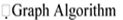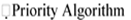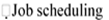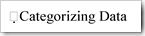23. Define priority queue with diagram and give the operations.

Priority queue is a data structure that allows at least the following two operations.

1. Insert-inserts an element at the end of the list called the rear.

2. DeleteMin-Finds, returns and removes the minimum element in the priority Queue.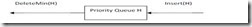Operations: Insert DeleteMin

24. Give the applications of priority queues.

There are three applications of priority queues

1. External sorting.

2. Greedy algorithm implementation.

3. Discrete even simulation.

4. Operating systems.

#### 1 comment:

1.Thanks For https://windowsroom.com/infix-pdf-editor/ You can also visit my Website windowsroom.com